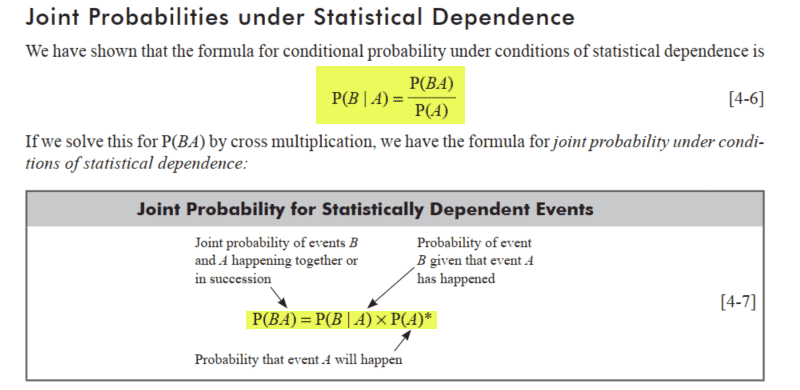# Conditional and joint probabilities of statistically dependent events

• I
• PainterGuy

#### PainterGuy

Hi,

If the events A and B are statically dependent then the following formulas are used to calculate conditional probability and joint probability but there is a problem. As I see it both formulas are dependent upon each other. One cannot calculate conditional probability without first calculating joint probability, and one cannot calculate joint probability without knowing condition probability! Where am I going wrong? Could you please help me? Thank you!•